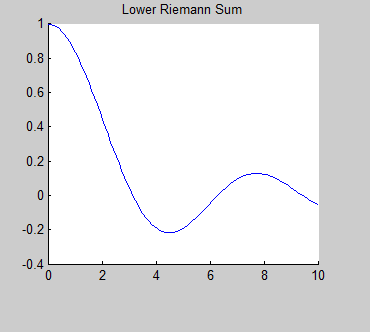Texas A&M University

This page contains links to papers and algorithms written by Jeremy Knight in conjunction with course taken from the graduate mathematics program at Texas A&M university.

## Projects/Research

• Applications of Finite Sets
This is Jeremy Knight's presentation link for the T.A.M.U. final oral exam for the Masters of Mathematics.
• Finite Element Method and the Poisson Problem
This is a link to web-pages, papers, and algorithms that I wrote to explain and demonstrate the use of applying the Finite Element Method to finding solutions of the Poisson Problem.
• Numerical Solutions of the Navier-Stokes Equations.
This is a project that I am currently developing to create a rigorous Matlab algorithm for finding numerical solutions to the Navier-Stokes equations at low Raleigh's numbers.
• Three Body Problem
The three body problem is a dynamical system problem modeling the chaotic path of three orbiting bodies affected by Newton's laws of gravity.
• Introduction to Rieman Sums
A lesson for students to learn about the Riemann sums and explore them using RiemannSums2.m which produces the plot shown to the right.## Iterative and Numerical Methods

The following Maple algorithms employ iterative algorithms to acquire numerical approximations.

General algorithms

Approximations for roots and solutions to systems of equations

Differentiation and Integration Algorithms

Differential Equation Algorithms

Partial Differential Equation Algorithms

## Cryptography

The following are Maple algorithms used for cryptography applications

Fractal Cryptography project

## Chaos and Dynamical Systems

Projects(pdf):

The following are Matlab algor

ithms for applications in dynamical systems and chaos problems.

• mandleset.m - plots the Mandelbrot set for a given function f(z).
Begin by using function handle to enter function such as the standard f(z)=z^2+c which can be entered into Matlab using
f(z,c)=@(z,c) z^2+c.
• threebody_script.m ; threebody.m - plots the path of the 3-body problem described above.
Download both files to the same directory and run threebody_script.m. The second file contains the ODEs.
Example plot to the right.
• twobody_script.m ; twobody.m - plots the path of two bodies in space affected by gravitational pull.
Download both files to the same directory and run twobody_script.m. The second file contains the ODEs.
Example: twobody_script(100,[-.35,.5,.25,-.5],1e-2)
• lyapunov.m - calculates the Lyapunov exponent for a given set of 1-dimensional data to determine the chaotic nature of the data.

## Other Matlab

• Function Transformation GUI
A great tool for demonstrating how function families transform.